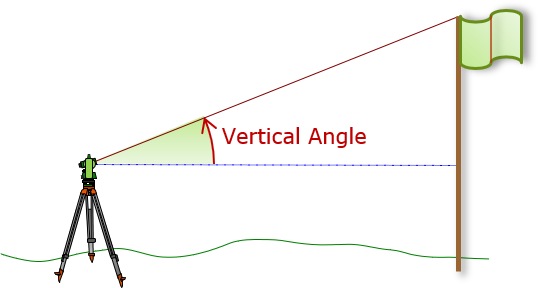## 2. Dimensions

### a. Distance

Distances in surveying may be measured in horizontal, vertical, or slope directions, Figure D-1.Figure D-1 Distances

Generally the result of a distance measurement is expressed either as a horizontal or vertical value.

In addition to orientation, a distance has a begin, end, and magnitude. All must be either expressed or implied by the type of distance to avoid ambiguity. For example when I stated my height:

• orientation: vertical implied

• begin: floor implied

• end: top of my head implied

• magnitude: 5’11”

### b. Angle

Angles are measured in a vertical or horizontal direction, Figure D-2; slope angles aren’t intentionally measured.(a) Vertical(b) Horizontal Figure D-2 Angles

Along with its orientation, an angle has four parts, Figure D-3:

 Start Direction Magnitude EndFigure D-3 Angle Parts